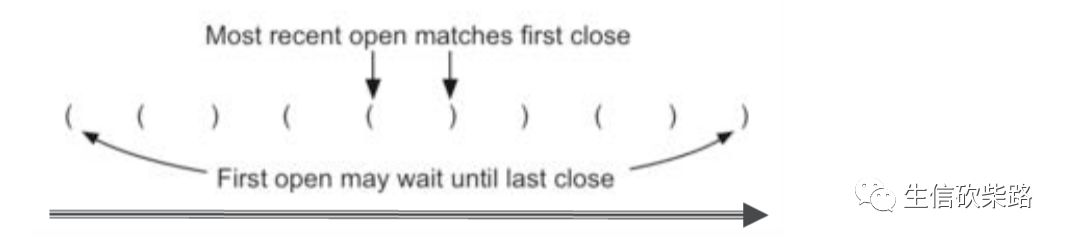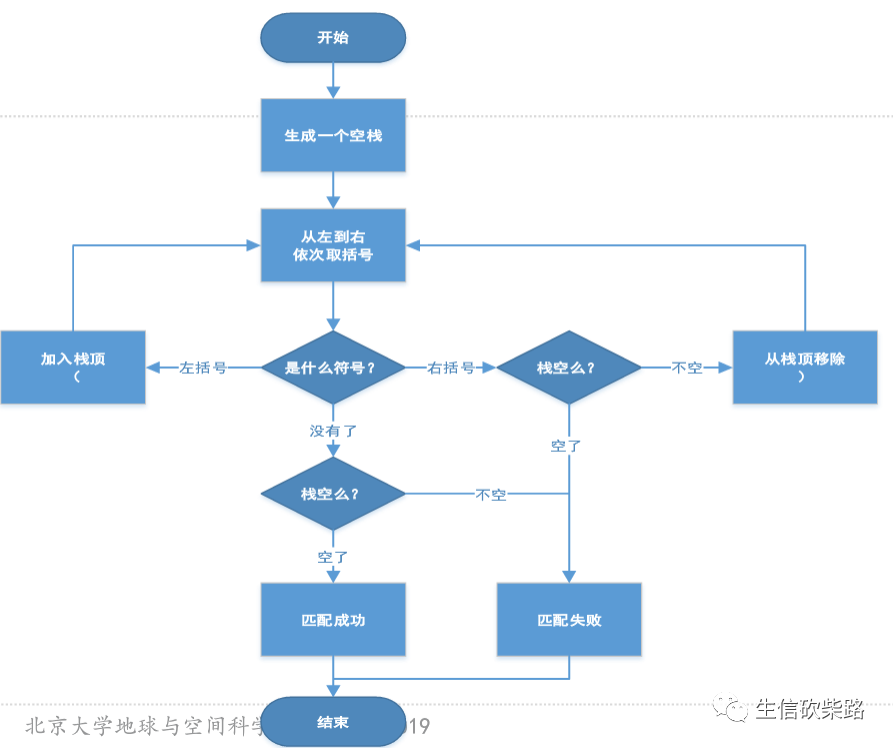# 数据结构与算法 笔记_数据结构与算法笔记—栈

``## 使用列表的末尾作为栈顶## 也可使用列表的开头作为栈顶，其实这两种方法的性能是不同的## 列表开头作为栈顶，复杂度为 O(n)## 列表末尾作为栈顶，复杂度为 O(1)class Stack:    def __init__(self):        self.items = []            def isEmpty(self):        return self.items == []            def push(self, item):        self.items.append(item)            def pop(self, item):        return self.items.pop()            def peek(self):        return self.items[len(self.items) - 1]            def size(self):        return len(self.items)````## Stack()是上面写好的类def matches(open, close):    opens = '([{'    closes = ')]}'    return opens.index(open) == closes.index(close)def check(strings):    s = Stack()    balance = True    index = 0    while index and         symbol = strings[index]        if symbol in '([{':            s.push(symbol)        else:            if s.isEmpty():                balance = False            else:                top = s.pop()                if not matches(top, symbol):                    balance = False        index += 1    if balance and s.isEmpty():        return True    else:        return False        ``